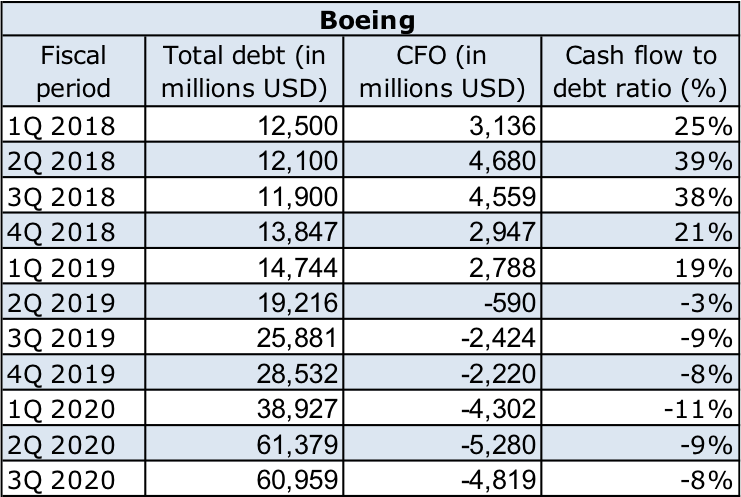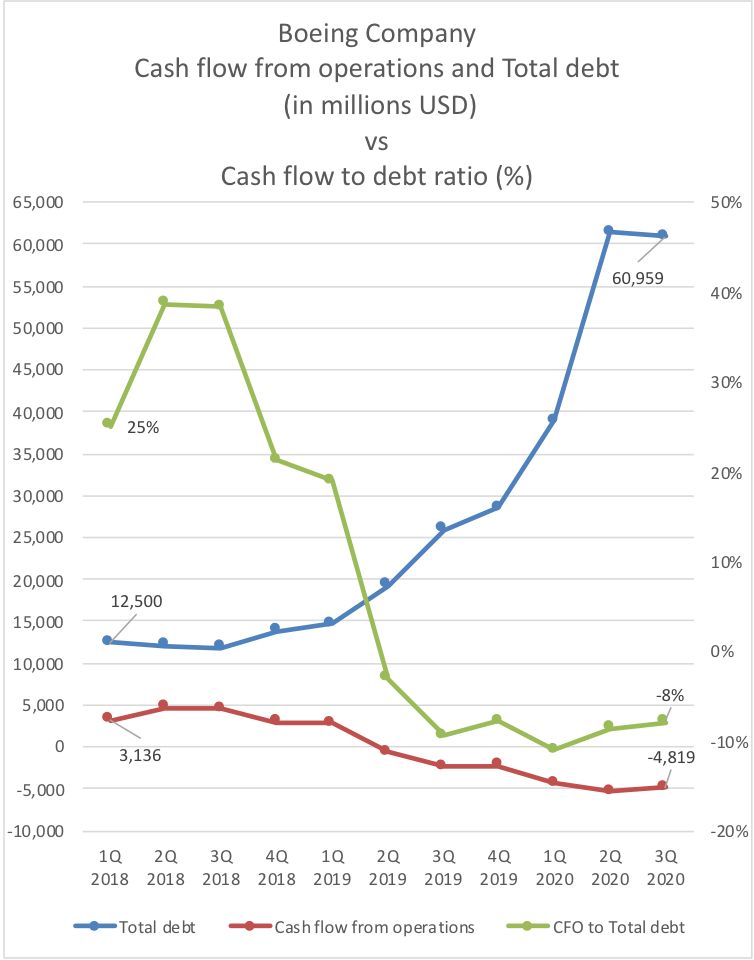# Cash Flow to Debt Ratio Calculator

By Arturo Barrantes
Last updated: Aug 12, 2021

Cash flow to debt ratio calculator is a coverage ratio tool that measures how much indebted a company is relative to the cash flow generated from operations during each fiscal period. This article will cover what is cash flow to debt ratio, its interpretation from an investor point of view, and a real example of cash flow to debt ratio analysis. You don't want to miss it!

## What is the cash flow to debt ratio?

Cash flow to debt ratio is a coverage ratio used to measure how capable a company covers its total debt. Similar to the interest coverage ratio, it indicates how healthy is a company.

This ratio uses cash flow from operations (CFO) instead of net income because the former one is considered to be a more clear representation of the real earnings a company produces.

As explained in the free cash flow calculator article, net income is affected by several non-cash items such as depreciation and amortization. Besides, it does not take into account cash flows from changes in working capital like increase or decrease in inventory levels.

Investors might consider that is more accurate to use EBITDA or EBIT; however, none of them take into account deferred income related to future contracts. Consequently, the ratio would probably be more alarming than reality, making us fall into an early misjudgment. That is why it is important to manage a ratio that compares real net cash inflow with total debt.

## How to calculate cash flow to debt ratio?

The cash flow to total debt formula has two components that combine as follows:

`Cash flow to debt ratio = Operating cash flow / Total debt`

You can quickly obtain the cash flow from operations figure from the cash flow statement; meanwhile, you can typically find the total debt in the quarter/annual report's financial highlights section. In case you are having problems obtaining the total debt, you can get it by the following sum:

`Total debt = Short term debt + Long term debt`

The short-term debt will always be in the current liabilities section of the balance sheet, while the long-term debt will be in the non-current liabilities section.

## Cash flow to debt ratio analysis

For a proper cash flow to debt ratio interpretation, we need to cover several fiscal periods. Then, we will have to analyze the trend and evaluate accordingly to one of the following scenarios:

• Operating cash flow increasing, total debt increasing: Cash flow to debt ratio will probably maintain itself, oscillating between specific values. In such cases, you are advised to evaluate a more extended period of time to better understand the trend or understand the capital structure of your company with the debt to capital ratio.

• Operating cash flow increasing, total debt decreasing: Cash flow to debt ratio will likely increase, and it is a good sign of positive company evolution. Dividends may start to be payed or even to be increased.

• Operating cash flow decreasing, total debt increasing: Cash flow to debt ratio will increase quickly. We do not recommend investing in such companies. If you are already in, you should consider selling your positions. Better to use our stock profit calculator to see how much you will get/loose for such investment, considering broker commissions.

• Operating cash flow decreasing, total debt decreasing: Cash flow to debt ratio may keep itself stable. However, we consider that the only good investment you should have is the one that is showing positive growth in operating cash flow. We recommend you to sell your positions; market capitalization will likely start to fall soon.

## Total debt to cash flow ratio formula

The debt to cash flow ratio is simply the reciprocal of the cash flow to total debt formula. You can interpret it as how many times the cash flow is contained in the total debt. The bigger this number means the company has more debt; thus, it may be less able to cover it.

Click on the advanced mode button in our nice cash flow to debt ratio calculator after adding the operating cash flow and total debt data to get this value. The result will be there already.

The total debt to cash flow ratio formula is as follows:

`Total debt to cash flow ratio = Total debt / Cash flow from operations`

## Cash flow to debt ratio example and analysis

On this occasion, we are going to review Boeing (NYSE: BA). Considering its 4Q 2018 quarter report, we have:

`Operating cash flow = 2,947 million USD`

`Total debt = 13.8 billion USD`

`Cash flow to debt ratio = 21.36 %`

`Debt to cash flow = 4.68`

Then, by taking into account the next quarter report (1Q 2019),

`Operating cash flow = 2,788 million USD`

`Total debt = 14.7 billion USD`

`Cash flow to debt ratio = 18.97 %`

`Debt to cash flow = 5.27`

We can clearly see the cash flow to debt ratio deteriorating from 21.36% to 18.97% from quarter to quarter. Besides, the debt to cash flow ratio increased from 4.68 to 5.27. That is a clear warning alarm.

Three months after, in the following quarter (2Q 2019 report) we even get a negative operating cash flow (-590 million USD) and a total debt equal to 19.2 billion USD. In fact, the total debt, in that quarter, had a compound annual growth rate of 58.8% if we compare it with the 2Q 2018 values.

Fast-forwarding to the present, after the 3Q 2020 report release, where the debt reached 61 billion USD, the market capitalization plunged to 93 billion USD, half of the market cap of 180 billion USD reached in 4Q 2018.

An investor who would have used our life-saving cash flow to debt ratio calculator would have been able to cover himself from a loss of 50% in the returns of his investments.

Here the mentioned data including other fiscal period values are presented:and, if we build a graph based on this results:We can clearly notice that the 2018 3rd quarter is the starting point of the divergence, then the cash flow from operations (red line) starts to decline, and the total debt (blue line) starts to rise. We also notice how the cash flow from operations (CFO) to total debt (green line) starts to decrease rapidly.

In conclusion, coverage ratios can warn us when a company might have a crash. A cautious investor would have sold its investment probably during the 2018 4th quarter.

Of course, it would have been crucial to have some room for maneuver in that situation, a factor of safety, meaning that you bought the stock when it was undervalued or at least fair priced. For such a endeavor, we recommend you to check our other powerful financial calculators, specially the ones about valuation like discounted cash flow calculator and ebitda multiple calculator.

Arturo Barrantes
Operating cash flow
\$
Total debt
\$
Cash flow to debt ratio
%
People also viewed…

### Alien civilization

The alien civilization calculator explores the existence of extraterrestrial civilizations by comparing two models: the Drake equation and the Astrobiological Copernican Limits👽

### Home value US

Use the home value calculator to find home value on a given date relaying on the Case-Shiller home price index.

### Online marketing conversion

The online marketing conversion calculator allows you to simulate the whole process of online sales, from impressions to customers, and to calculate the ROI.

### Steps to calories

Steps to calories calculator helps you to estimate the total amount to calories burned while walking.